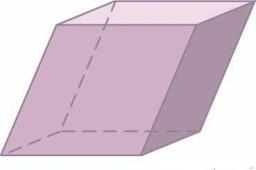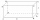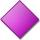# Prism

The base of the prism is a rhombus with a side 30 cm and a height 27 cm long.
The height of the prism is 180% longer than the side length of the rhombus.

Calculate the volume of the prism.

V =  68040 cm3

### Step-by-step explanation:Did you find an error or inaccuracy? Feel free to write us. Thank you!Tips to related online calculators
Do you want to convert length units?
Tip: Our volume units converter will help you with the conversion of volume units.

#### You need to know the following knowledge to solve this word math problem:

We encourage you to watch this tutorial video on this math problem:

## Related math problems and questions:

• Four prismsQuestion No. 1: The prism has the dimensions a = 2.5 cm, b = 100 mm, c = 12 cm. What is its volume? a) 3000 cm2 b) 300 cm2 c) 3000 cm3 d) 300 cm3 Question No.2: The prism base is a rhombus with a side length of 30 cm and a height of 27 cm. The height of t
• Cardboard boxWe want to make a cardboard box shaped quadrangular prism with rhombic base. Rhombus has a side of 5 cm and 8 cm one diagonal long. The height of the box to be 12 cm. The box will be open at the top. How many square centimeters cardboard we need, if we ca
• PrismCalculate the volume of the rhombic prism. The prism base is a rhombus whose one diagonal is 47 cm, and the edge of the base is 28 cm. The edge length of the base of the prism and height is 3:5.
• BoxCardboard box-shaped quadrangular prism with a rhombic base. Rhombus has a side 5 cm, and one diagonal 8 cm long, and the box's height is 12 cm. The box will open at the top. How many cm2 of cardboard do we need to cover overlap and joints that are 5% of
• Surface and volume od cuboidThe content area of the square base of the cuboid is Sp = 36 cm2, and its height is 80 mm. Determine its surface area and volume.
• The regularThe regular triangular prism has a base in the shape of an isosceles triangle with a base of 86 mm and 6.4 cm arms, the height of the prism is 24 cm. Calculate its volume.
• Triangular prismThe base of the perpendicular triangular prism is a right triangle with a leg length of 5 cm. The content area of the largest sidewall of its surface is 130 cm², and the height of the body is 10 cm. Calculate its volume.
• Rhombus baseCalculate the volume and surface area of prisms whose base is a rhombus with diagonals u1 = 12 cm and u2 = 15 cm. Prism height is twice the base edge length.
• Triangular prismThe base of the perpendicular triangular prism is a rectangular triangle with a hypotenuse of 10 cm and one leg of 8 cm. The prism height is 75% of the perimeter of the base. Calculate the volume and surface of the prism.
• The hollow cylinderThe hollow cylinder has a height of 70 cm, an outer diameter of 180 cm, and an inner diameter of 120 cm. What is the surface of the body, including the area inside the cavity?
• Triangular prismCalculate the volume and surface of the triangular prism ABCDEF with base of a isosceles triangle. Base's height is 16 cm, leg 10 cm, base height vc = 6 cm. The prism height is 9 cm.
• PrismCalculate the surface area and volume of a prism with a body height h = 10 cm, and its base has the shape of a rhomboid with sides a = 5.8 cm, b = 3 cm, and the distance of its two longer sides is w = 2.4 cm.
• Base sideIn a quadrilateral prism, are known surface area S = 12400 mm2 base side m = 40mm and prism height = 120mm, what is the length of base side n =?
• Rhombus 4The circumference of the rhombus is 44 cm, its height is 89 mm long. Calculate its content area.
• Cuboid aquariumCuboid 25 times 30 cm. How long is third side if cuboid contains 30 liters of water?The surface of the regular quadrilateral prism is 8800 cm2, and the base edge is 20 cm long. Calculate the volume of the prismThe box-shaped aquarium is 40 cm high; the bottom has dimensions of 70 cm and 50 cm. Simon wanted to create an exciting environment for the fish, so he fixed three pillars to the bottom. They all have the shape of a cuboid with a square base. The base edg